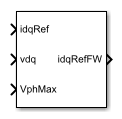# PMSM Field-Weakening Controller

Permanent magnet synchronous machine field-weakening controller

• Library:
• Simscape / Electrical / Control / PMSM Control

•## Description

The PMSM Field-Weakening Controller block implements a field-weakening controller for a permanent magnet synchronous machine (PMSM).

Use this block to enforce phase voltage constraints on a current-controlled PMSM. The block decreases the PMSM phase voltage by adjusting the angle of the reference current vector when the voltage vector magnitude exceeds its limit. The block does not adjust the amplitude of the current vector.

You can use this block as part of a PMSM control system:

• Use the zero d-axis control technique to generate an unconstrained current reference vector to drive the PMSM. You can implement this strategy with the PMSM Current Reference Generator block.

• Use this block to adjust the angle of the current reference vector in order to satisfy voltage phase constraints.

• Use a PMSM Current Controller to generate a voltage reference vector to drive the PMSM.

### Equations

An internal integral controller outputs a factor β∈[0,1], which is determined by how closely the required stator voltage approaches the saturated voltage value at any instant in time:

• When the required stator voltage exceeds the limit, β tends to 0, decreasing the q-axis current.

• When the required stator voltage is within its limit, β tends to 1 and the angle remains unchanged.

This diagram shows the structure of the field-weakening controller.In the diagram, you provide the modulation index threshold Mth as an input parameter to the block, and the block computes the modulation index M as the ratio between the actual phase voltage and the maximum available phase voltage Vph_max:

`$M=\frac{\sqrt{{v}_{d}^{2}+{v}_{q}^{2}}}{{V}_{ph_max}},$`

where vd and vq are the d-axis and q-axis components of the voltage vector.

## Ports

### Input

expand all

Desired d- and q-axis currents for control of permanent magnet synchronous motor, in A.

Data Types: `single` | `double`

Direct and quadrature axis voltages of permanent magnet synchronous motor, in V.

Data Types: `single` | `double`

Maximum allowable voltage in each phase, in V.

Data Types: `single` | `double`

### Output

expand all

Field-weakening reference direct and quadrature axis currents, in A.

Data Types: `single` | `double`

## Parameters

expand all

Reference modulation index.

Integrator gain of the field-weakening controller.

Anti-windup gain of the field-weakening controller.

Sample time for the block (-1 for inherited). If you use this block inside a triggered subsystem, set the sample time to `-1`. If you use this block in a continuous variable-step model, set the sample time explicitly.

 Wai, J., and T. M. Jahns. "A new control technique for achieving wide constant power speed operation with an interior PM alternator machine." In Industry Applications Conference. Vol. 2, 2001, pp. 807-814.